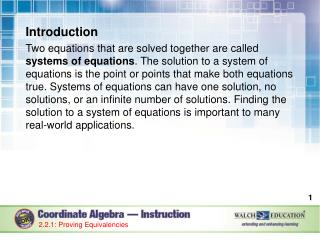DownloadDownload PresentationIntroduction

# Introduction

Download Presentation## Introduction

- - - - - - - - - - - - - - - - - - - - - - - - - - - E N D - - - - - - - - - - - - - - - - - - - - - - - - - - -
##### Presentation Transcript

1. Introduction Two equations that are solved together are called systems of equations. The solution to a system of equations is the point or points that make both equations true. Systems of equations can have one solution, no solutions, or an infinite number of solutions. Finding the solution to a system of equations is important to many real-world applications. 2.2.1: Proving Equivalencies

2. Key Concepts There are various methods to solving a system of equations. Two methods include the substitution method and the elimination method. 2.2.1: Proving Equivalencies

3. Key Concepts, continued Solving Systems of Equations by Substitution This method involves solving one of the equations for one of the variables and substituting that into the other equation. 2.2.1: Proving Equivalencies

4. Key Concepts, continued 2.2.1: Proving Equivalencies

5. Key Concepts, continued Solutions to systems are written as an ordered pair, (x, y). This is where the lines would cross if graphed. If the resulting solution is a true statement, such as 9 = 9, then the system has an infinite number of solutions. This is where lines would coincide if graphed. 2.2.1: Proving Equivalencies

6. Key Concepts, continued If the result is an untrue statement, such as 4 = 9, then the system has no solutions. This is where lines would be parallel if graphed. Check your answer by substituting the x and y values back into the original equations. If the answer is correct, the equations will result in true statements. 2.2.1: Proving Equivalencies

7. Key Concepts, continued Solving Systems of Equations by Elimination Using Addition or Subtraction This method involves adding or subtracting the equations in the system so that one of the variables is eliminated. Properties of equality allow us to combine the equations by adding or subtracting the equations to eliminate one of the variables. 2.2.1: Proving Equivalencies

8. Key Concepts, continued 2.2.1: Proving Equivalencies

9. Key Concepts, continued Solving Systems of Equations by Elimination Using Multiplication This method is used when one set of variables are neither opposites nor the same. Applying the multiplication property of equality changes one or both equations. Solving a system of equations algebraically will always result in an exact answer. 2.2.1: Proving Equivalencies

10. Key Concepts, continued 2.2.1: Proving Equivalencies

11. Common Errors/Misconceptions finding the value for only one of the variables of the system forgetting to distribute negative signs when substituting expressions for variables forgetting to multiply each term by the same number when solving by elimination using multiplication 2.2.1: Proving Equivalencies

12. Guided Practice Example 2 Solve the following system by elimination. 2.2.1: Proving Equivalencies

13. Guided Practice: Example 2, continued Add the two equations if the coefficients of one of the variables are opposites of each other. 3y and –3y are opposites, so the equations can be added. Add downward, combining like terms only. 2.2.1: Proving Equivalencies

14. Guided Practice: Example 2, continued Simplify. 3x= 0 2.2.1: Proving Equivalencies

15. Guided Practice: Example 2, continued Solve the equation for the second variable. 2.2.1: Proving Equivalencies

16. Guided Practice: Example 2, continued Substitute the found value, x = 0, into either of the original equations to find the value of the other variable. 2.2.1: Proving Equivalencies

17. Guided Practice: Example 2, continued The solution to the system of equations is . If graphed, the lines would cross at . ✔ 2.2.1: Proving Equivalencies

18. Guided Practice: Example 2, continued 2.2.1: Proving Equivalencies

19. Guided Practice Example 3 Solve the following system by multiplication. 2.2.1: Proving Equivalencies

20. Guided Practice: Example 3, continued Multiply each term of the equation by the same number. The variable x has a coefficient of 1 in the first equation and a coefficient of –2 in the second equation. Multiply the first equation by 2. 2.2.1: Proving Equivalencies

21. Guided Practice: Example 3, continued Add or subtract the two equations to eliminate one of the variables. 2.2.1: Proving Equivalencies

22. Guided Practice: Example 3, continued Simplify. 2.2.1: Proving Equivalencies

23. Guided Practice: Example 3, continued The system does not have a solution. There are no points that will make both equations true. ✔ 2.2.1: Proving Equivalencies

24. Guided Practice: Example 3, continued 2.2.1: Proving Equivalencies